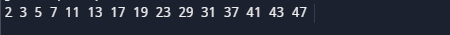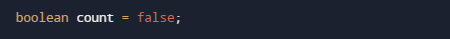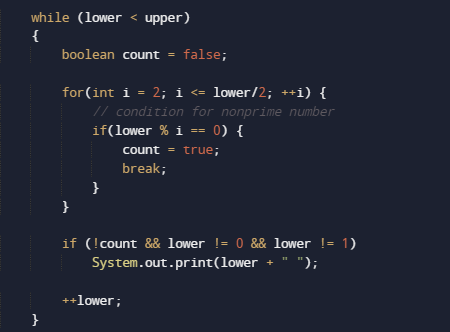# Java Program to Print Prime Numbers

In this tutorial you will learn about the Java Program to Print Prime Numbers and its application with practical example.

In this tutorial, we will learn to create a Java program that will print Prime numbers between given number using Java programming.

## Prerequisites

Before starting with this tutorial we assume that you are best aware of the following Java programming topics:

• Java Operators.
• Basic Input and Output
• Class and Object.
• Basic Java programming.
• If-else Statements.
• Loops in Java
• While and for Loop.

## What Is Prime number?

Any positive Number which is bigger than 1 and it can only  divisible by itself and by 1 and it has maximum of factors is two is called as Prime number.Rather we can say that a prime number is a number which only divided by him or 1.

## Java Program to Print Prime Numbers.

In our program we will find that Prime number between the number provided by user using while loop.Firstly we declare required header file and variable and also initiates required values in variable. Next we take value from user at run time and find is prime number between given number.

## OutputIn the above program, we have first declared and initialized a set variables required in the program.

• upper= it will hold upper range
• lower= it will hold lower range.
• i = for iteration.
• count = will give us number is prime or not.

And  in the next statement values will be  assigned to variables “upper” and “lower”. And at start value of count set to false default.After getting the values in  a program we start the loop within the range from lower to upper and find prime number between them.whenever value of count became “true” means the value of a number is completely dived by itself,then we print the number that divide itself and not by others is a prime number .Then the next number in the loop is checked, till all numbers are checked within the range of lower an upper as  we taken.So here the result shows prime numbers within  the  range lies are.

In this tutorial we have learn about the Java Program to Print Prime Numbers and its application with practical example. I hope you will like this tutorial.